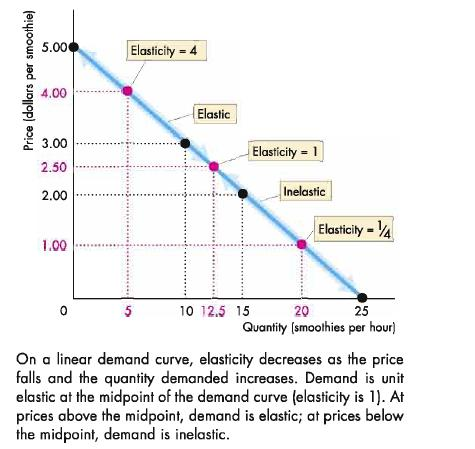# Straight line (linear) demand curve

A straight line demand curve will have a different elasticity at each point on it.

The price elasticity of demand can also be measured at any point on the demand curve.

If the demand curve is linear (straight line), it has a unitary elasticity at the midpoint. The total revenue is maximum at this point. Any point above the midpoint has elasticity greater than 1, (Ed > 1).

Here, price reduction leads to an increase in the total revenue (expenditure). Below the midpoint elasticity is less than 1. (Ed < 1). Price reduction leads to reduction in the total revenue of the firm. Now the question arises, why does a straight line demand curve have different elasticity at each point? The value of PED falls as price falls.

The reason is that low priced products have a more inelastic demand than high priced products, because consumers are not that price sensitive when the product is inexpensive, Similarly the value of PED is higher when the prices increase because consumers are more sensitive to price change when the good is expensive. A mathematical explanation can be given as follows. As we seen in diagram below